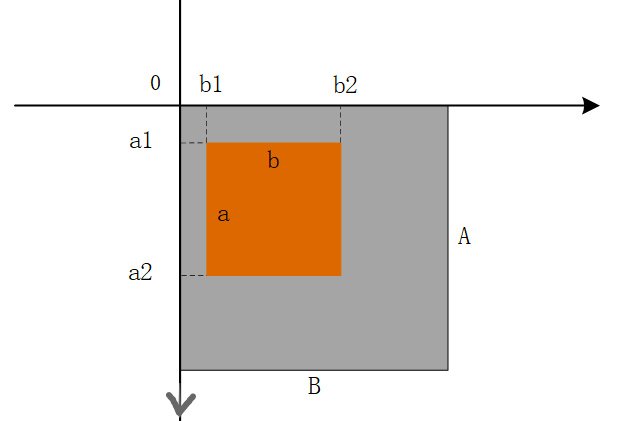## 使用PIL处理图片

### 0. 加载模块

``from PIL import ImageFont, ImageDraw, Image``

### 1. 图片对象基本处理

``img = Image.open('./file/pic01.png')  # 加载图片对象img.format  # 获取图片类型(后缀)img.size  # 获取图片尺寸img.mode  # 获取图片模式 如 RGBimg.info  # 获取图片信息字典``

### 2. 改变图像的模式

``img2 = img.convert('RGBA')``

### 3.  新建图层

``# 新建一个图层# 模式为 'RGB'# 尺寸大小为 (256, 256)# 三通道颜色值为 (128, 128, 128)im = Image.new('RGB', (256, 256), (128, 128, 128))``

### 4.  图像复制&粘贴

``# 新建图层 im1im1 = Image.new('RGB', (256, 256), (128, 128, 128))# 新建图层 im2im2 = Image.new('RGB', (128, 128), (0, 28, 128))# 复制一个图层 im3im3 = im2.copy()# ################################################## 在图层 im1 上粘贴图层 im2# 粘贴位置坐标为 (100, 100) -- 默认为左上角为原点Oim1.paste(im2, (100, 100))``

4.1 拷贝图片区域box``box = (b1, a1, b2, a2)  # 确定拷贝区域坐标box_copy = im.crop(box)  # 将im表示的图片对象拷贝到 box_copy 中, 大小为box``

### 5. 分离出图像的各通道数据(元组)

``# 假设 box_copy 的模式为 'RGB'r, g, b = box_copy.split()r.mode  # 分离后的模式都为 'L'r.size  # 分离后size不变``

### 6. 获取图像的非零坐标

``# 假设图片 pic1.png 的尺寸为 (400, 400)im = Image.open('./pic1.png')im_tuple = im.getbbox()  # im_tuple 值为 (0, 0, 400, 400)``

### 7. 图像改变尺寸

``im.size  # im图片对象的尺寸为(2560, 1440)im1 = im.resize((1000, 1000))  # 将im 的尺寸更改为(1000, 1000)im1.size  # im1 图片对象的尺寸为(1000, 1000)``

### 8. 图片保存

``# 说明：    # 该方法返回为空    # pic_file -- 图片文件保存路径已经文件名称    # quality -- 图片保存质量 默认为 100 设置该参数可以对目标文件字节数进行调整img.save(pic_file, optimize=True, quality=quality_num)``

### 9. 图片添加文字

``word_font = './SourceHanSansCN-Normal.ttf'  # 指定某字体类型word_size = 38  # 指定字体大小# 加载并创建一个字体对象,从指定的文件加载了一个字体对象, 并为指定大小的字体创建了字体对象font = ImageFont.truetype(word_font, word_size)  # 利用img 创建一个可以在给定图像上绘图的对象drawdraw = ImageDraw.Draw(img) # 使用对象 draw 在 img 上添加指定文字    # font 指定字体对象    # fill 指定文字颜色word_loc = (100, 100)  # 文字位置 tupleword_str = '这是文字'  # 文字内容 strfill = 'red'draw.text(word_loc, word_str, font=font, fill=fill)``